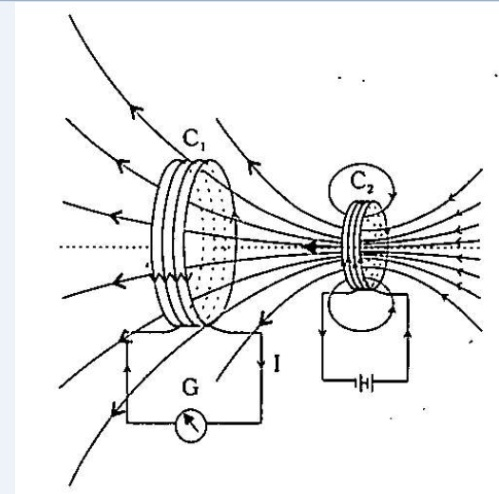Home/Class 12/Physics/

## QuestionPhysicsClass 12Current is induced in coil $$C_{1}$$ due to motion of the current carrying coil $$C_{2}$$.
In Fig. the bar magnet is replaced by a second coil $$\mathrm{C}_{2}$$ connected to a battery. The steady current in the coil $$\mathrm{C}_{2}$$ produces a steady magnetic field. As coil $$\mathrm{C}_{2}$$ is moved towards the coil $$C_{1}$$, the galvanometer shows a deflection. This indicates that electric current is induced in coil $$\mathrm{C}_{1}$$. When $$\mathrm{C}_{2}$$ is moved away, the galvanometer shows a deflection again, but this time in the opposite direction. The deflection lasts as long as coil $$\mathrm{C}_{2}$$ is in motion. When the coil $$\mathrm{C}_{2}$$ is held fixed and $$\mathrm{C}_{1}$$ is moved, the same effects are observed. Again, it is the relative motion between the coils that induces the electric current.
What would you do to obtain a large deflection of the galvanometer?
How would you demonstrate the presence of an induced current in the absence of a galvanometer?

See analysis below
4.64.6## Solution

To get large deflection in the galvanometer, one can
$$(i)$$ Increase the relative speed between $$C_1$$ and $$C_2$$
$$(ii)$$ Increase the number of turns of coil $$C_1$$
$$(iii)$$ Insert  the medium of greater relative permeability to enhance the magnetic field inside the coil $$C_1$$
⟹ We can use either a motor or connect a light source like ordinary bulb. So due to phenomenon  of electromagnetic induction, the glow of bulb or movement of rotor will indicate the presence of induced current in the circuit.